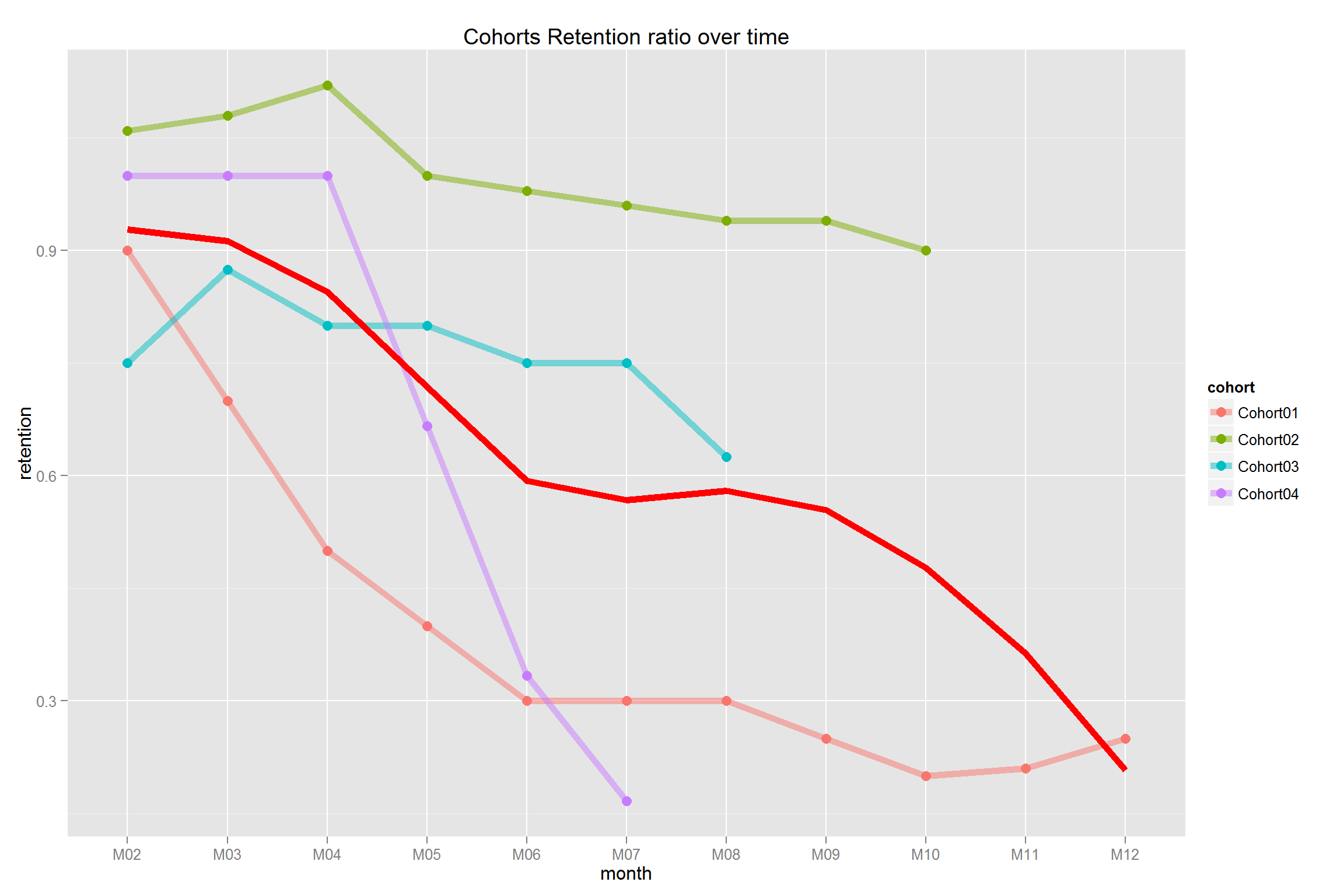In any business we really want to keep hold of our customers. We all know that customers come and go. A good measurement is Customer Retention. We can count customers in a particular month and divide by the customers at the start. For example after a large marketing campaign we recruited 2000 customers, 6 months later the customer count was 1700 we say our retention rate is 85%.

This is bread and butter for anyone analysing customers. What is really interesting to see is how the retention rate has changed over time for the different customer groups.

We can argue the longer we keep customers the higher level of loyalty we have. Of course, this could be inertia or because we have a great brand/service.

We can use R to analyse this. I have to thank Sergey Bryl’ for his extensive writing on marketing analytics.

First we have to load sample data.

#Sample Data

cohort.clients <- data.frame(cohort=c('Cohort01','Cohort02',
'Cohort03','Cohort04'),
M01=c(1000,0,0,0),
M02=c(900,0,0,0),
M03=c(700,500,0,0),
M04=c(500,530,0,0),
M05=c(400,540,400,0),
M06=c(300,560,300,300),
M07=c(300,500,350,300),
M08=c(300,490,320,300),
M09=c(250,480,320,300),
M10=c(200,470,300,200),
M11=c(210,470,300,100),
M12=c(250,450,250,50))

This data shows the number of customer cohorts (groups) over time.

##     cohort  M01 M02 M03 M04 M05 M06 M07 M08 M09 M10 M11 M12
## 1 Cohort01 1000 900 700 500 400 300 300 300 250 200 210 250
## 2 Cohort02    0   0 500 530 540 560 500 490 480 470 470 450
## 3 Cohort03    0   0   0   0 400 300 350 320 320 300 300 250
## 4 Cohort04    0   0   0   0   0 300 300 300 300 200 100  50

A little data cleaning is required to remove 0’s and align the cohorts to M1. This ensures the plot lines are aligned left which makes for easier reading.

library(dplyr)
library(ggplot2)
library(reshape2)

cohort.clients.r <- cohort.clients #create new data frame
totcols <- ncol(cohort.clients.r) #count number of columns in data set

for (i in 1:nrow(cohort.clients.r)) { #shifting each row
df <- cohort.clients.r[i,] #select row from the data frame
df <- df[ , !df[]==0] #remove columns with zeros
partcols <- ncol(df) #count number of columns in a row (<>zeros)

#fill columns after values by zeros
if (partcols < totcols) df[, c((partcols+1):totcols)] <- 0
cohort.clients.r[i,] <- df #replace initial row by new one
}

x <- cohort.clients.r[,c(2:13)]
y <- cohort.clients.r[,2]
reten.r <- apply(x, 2, function(x) x/y )
reten.r <- data.frame(cohort=(cohort.clients.r\$cohort), reten.r)

#charts
reten.r <- reten.r[,-2] #remove M01 data because it is always 100%

Having prepared the data it’s straightforward to plot with ggplot2.

#retention chart
cohort.chart1 <- melt(reten.r, id.vars = 'cohort')
colnames(cohort.chart1) <- c('cohort', 'month', 'retention')
cohort.chart1 <- filter(cohort.chart1, retention != 0)
p <- ggplot(cohort.chart1, aes(x=month, y=retention, group=cohort, colour=cohort))
p + geom_line(size=2, alpha=1/2) +
geom_point(size=3, alpha=1) +
geom_smooth(aes(group=1), method = 'loess', size=2, colour='red', se=FALSE) +
labs(title="Cohorts Retention ratio over time")I like this chart as we can visually understand what is happening to customers over time. What is going on with Cohort 4? Oh, after looking we find this group represents Young Males (16-22). Our marketing wizards are going to have do something special to sort this one.